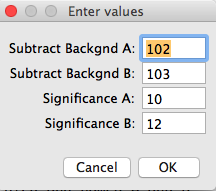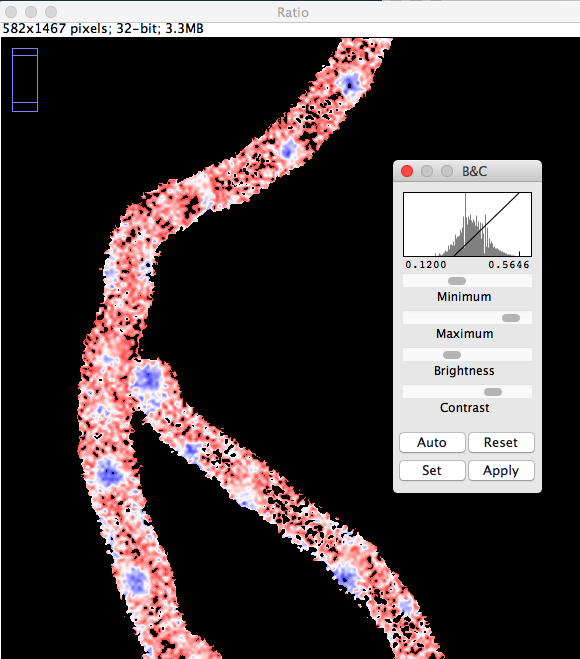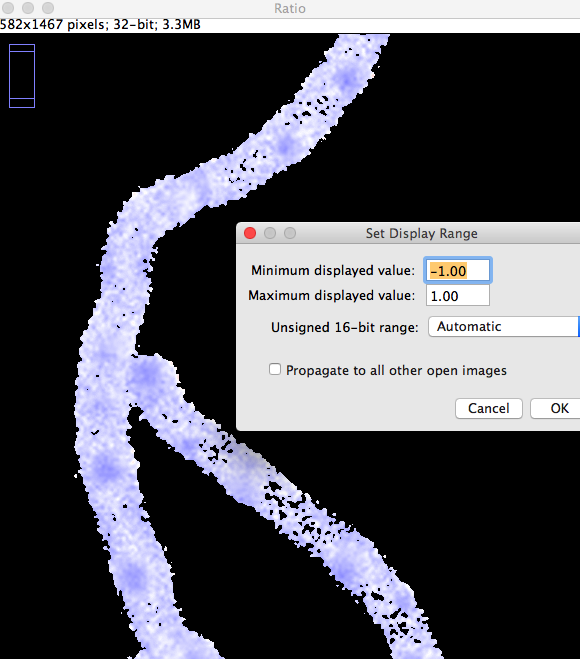### Using ImageJ to show "Generalised Polarisation" (GP)

N.Vischer
10-jun-2016

This ImageJ macro visualizes the different fluorescence distribution on two images using the equation:
output = (A-B)/(A+B)
The result is shown using a color LUT red-white-blue.
- Red: image B dominates
- White: neutral
- Blue: image A domiates

1. Make sure that only two images were opened in correct order A-B (see Window menu)

2. Install macro CalculateGP02.txt

**The following actions are performed: **

• If more than 2 images are open, these will be deleted after confirmation
• A dialog box is presented to enter 4 values: background subtraction and significance [see suggestion (a) below]
• the two source images are duplicated and named A and B
• from image A, background is subtracted (demo: value 102)
• from image B, background is subtracted (demo: value 103)
• in image A, non-significant pixels (demo value: <= 10) are set to zero
• in image B, non-significant pixels (demo value: <= 12) are set to zero
(non-significant pixels are considered to be not part of the cell)

• image "A-B" is calculated as 32 bit

• image "A+B" is calculated as 32 bit

• image "Ratio" is calculated from (A-B)/(A+B) as 32 bit

• in image "Ratio", all values <= -1 and >= 1 are set to NaN (not a number) Such a pixel has been zero in one or both channels after background and and significance process

• a lookup table is created with gradient red-white-blue corresponding to indices 0-128-255. However, both end points are set to black.

• min and max display values are set to -1 to 1. This means that the lookup table is applied symmetrically, e.g.
-0.99 = red
0 = white
0.99 = blue

• you can change this range by choosing menu Image>Adjust> Brightness&Contrast, then click on "Set" and change the values (don't click Apply).

• alternatively, do this via macro command: the macro "Set LUT example" applies an asymmetric range -0.4 to 0.8, i.e. 0.2 appears white

• For viewing purposes, you can duplicate the scientific 32bit image and convert it to RGB via menu Image>Type>RGBcolorFig 1: User values for background and significance
(see suggestion [a] below)Fig 2: Change sliders Min and Max of Display Range via Image>Adjust>Brightess/ContrastFig 3: Change number fields Min and Max of Display Range

[a] Suggestion for background subtraction and significance:
In either of the two images, you could choose